# Complete the following reaction sequence. giving details of all Complete the following reaction sequence. giving details...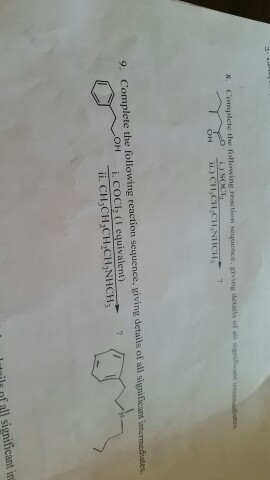Complete the following reaction sequence. giving details of all Complete the following reaction sequence. giving details of all significant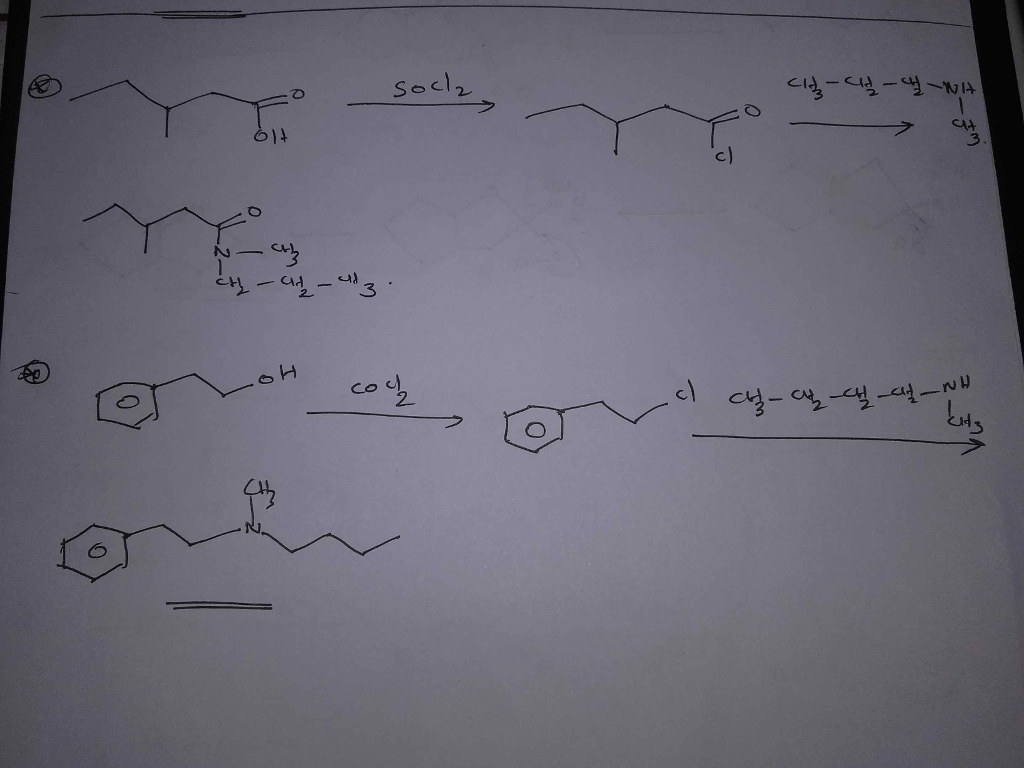#### Earn Coin

Coins can be redeemed for fabulous gifts.

Similar Homework Help Questions
• ### Complete the following reaction scheme, giving complete stereochemic details as relevant. Explain please! Complete the following...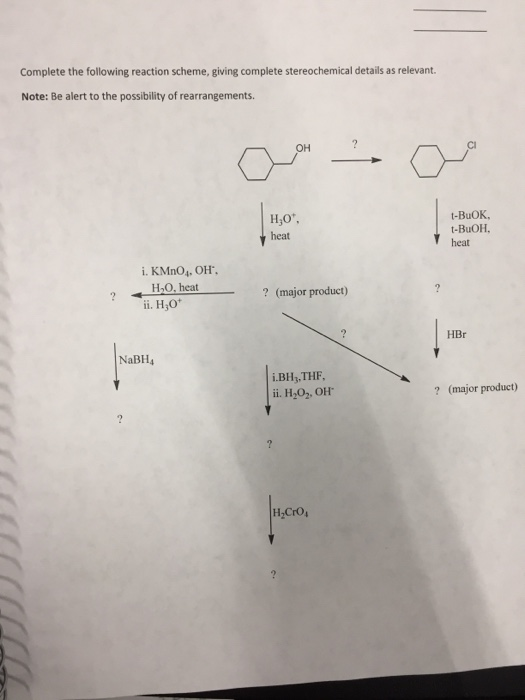Complete the following reaction scheme, giving complete stereochemic details as relevant. Explain please! Complete the following reaction scheme, giving complete stereochemical details as relevant. Note: Be alert to the possibility of rearrangements OH t-BuOK, t-BuOH. heat heat i. KMnO4. ОН. 2 120,heat? (major product) ? (major product) + ii. H,0 HBr NaBH4 i.BHy,THF ii. H202. OH ? (major product)

• ### Last Name First Name Student I.D. number Problem 3 (10 pts) Complete the following reaction sequence,...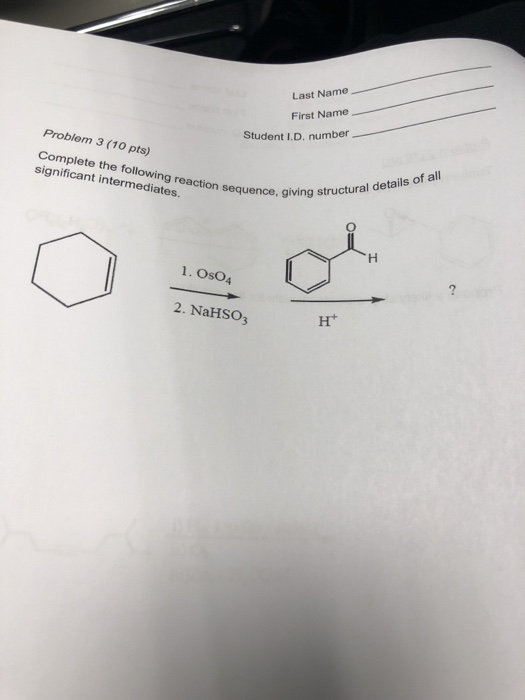Last Name First Name Student I.D. number Problem 3 (10 pts) Complete the following reaction sequence, g significant intermediates. giving structural details of all 1. OsO4 2. NaHSO3 Ht

• ### what is the final product of the following reaction sequence? Give structural details of all significant...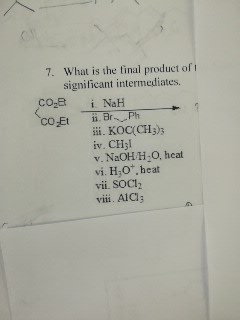what is the final product of the following reaction sequence? Give structural details of all significant intermediates. What is the tinal product of significant intermediates. 7. co i NaH ii. KOC(CH3)s iv. C1131 v. NaOHH-O, heat vi. H30", heat vii. SOCI vii. AlCI

• ### please answer i,ii,iii,iv,v Predict the major product(s) of the following reaction, giving regiochemical and/or stereochemical details...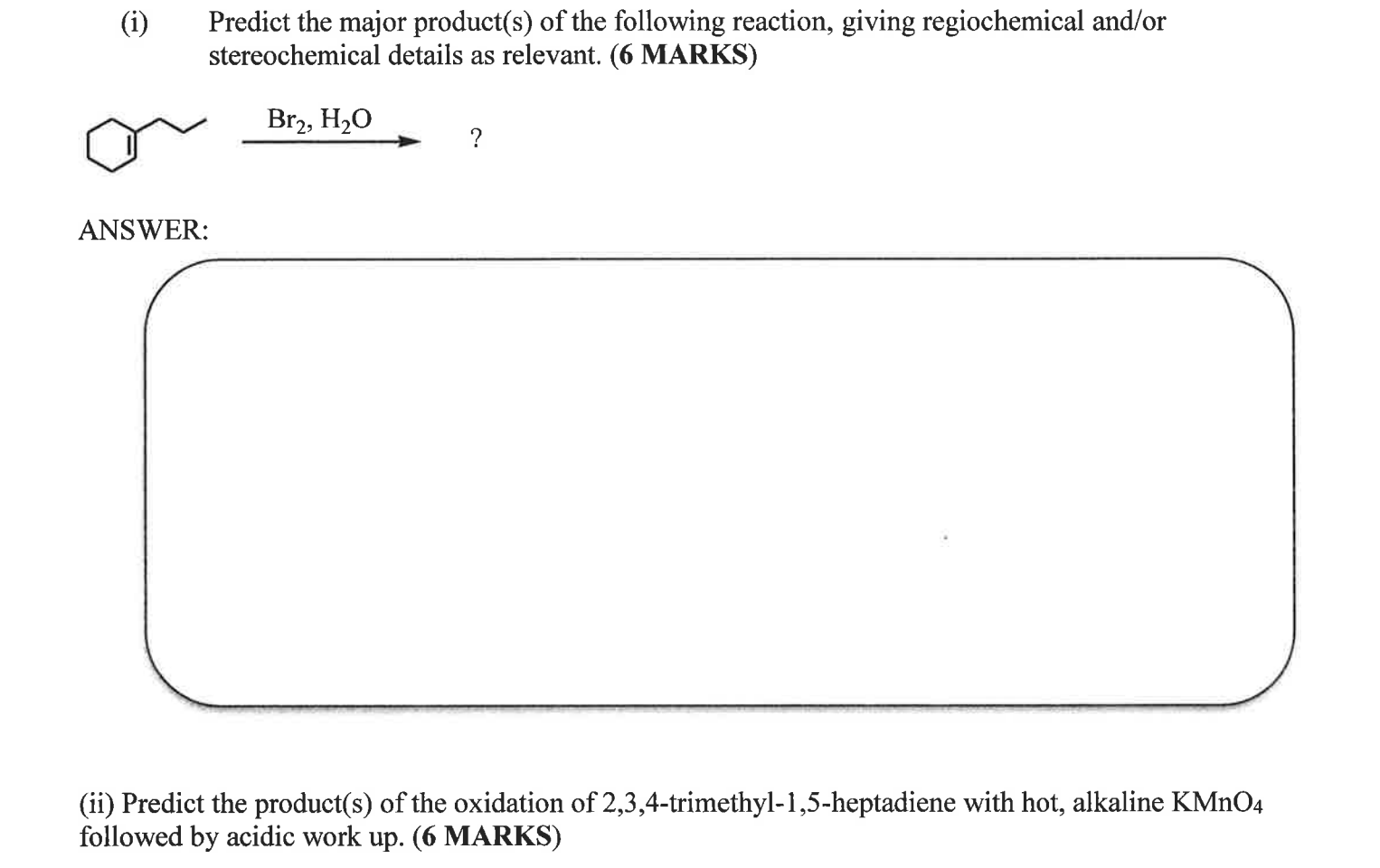please answer i,ii,iii,iv,v Predict the major product(s) of the following reaction, giving regiochemical and/or stereochemical details as relevant. (6 MARKS) q Bry, H0 ? ANSWER: (ii) Predict the product(s) of the oxidation of 2,3,4-trimethyl-1,5-heptadiene with hot, alkaline KMnO4 followed by acidic work up. (6 MARKS) (iii) Complete the following reaction sequence, giving structures for compounds C and D (Na2CO3 just acts as a base!): CH,SOCI CH,OH Na2CO3 D DMF (6 MARKS) D (iv) Supply the missing structures A and B....

• ### 3. (6 pts) What is the final product of the following reaction sequence? Give structural details...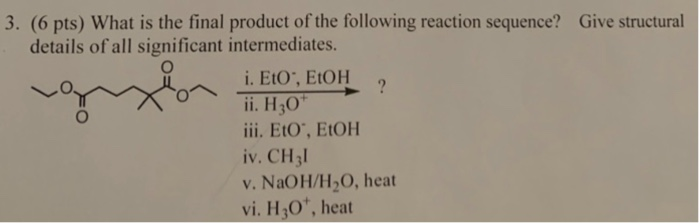3. (6 pts) What is the final product of the following reaction sequence? Give structural details of all significant intermediates. i. Eto, EtOH i. H30 ili. Eto, EtOH iv. CH3l v. NaOH/H2O, heat vi. H3O, heat

• ### 16. What is the final product of the following reaction sequence? Give structural details of all...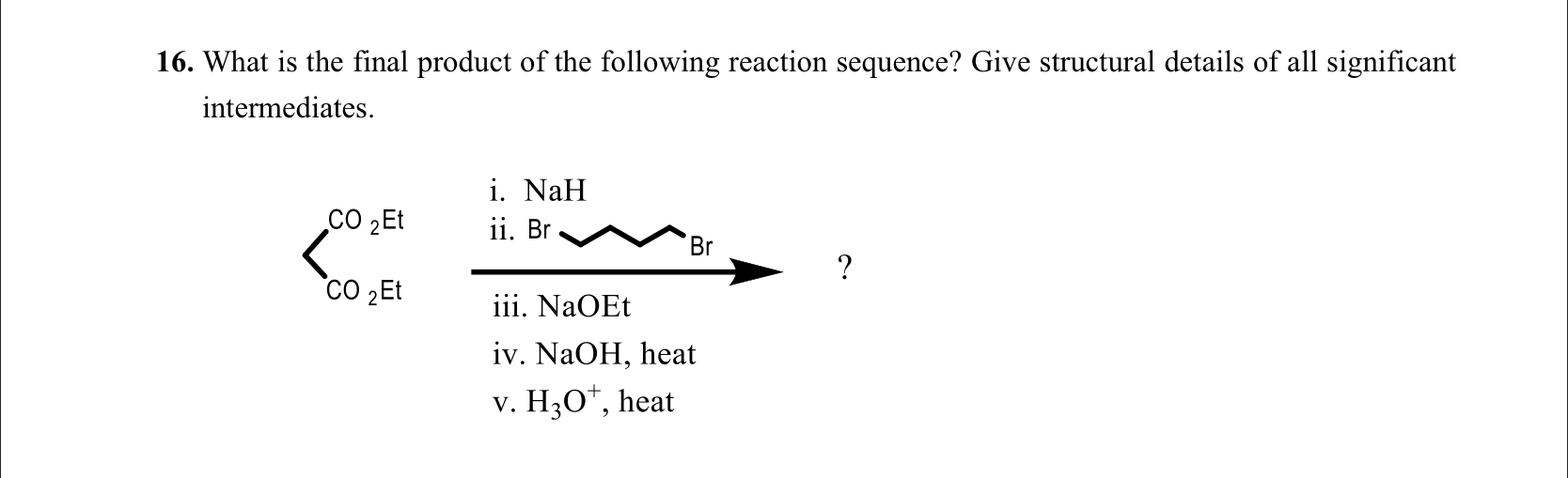16. What is the final product of the following reaction sequence? Give structural details of all significant intermediates. i. NaH ii. Br CO 2Et CO 2Et Br iii. NaOEt iv. NaOH, heat v. H2O7, heat Part I. Multiple Choice, select best answer. 1. In the molecule below, which of the hydrogens are the most acidic? II IV sonryer i II í V (a) 1 (b) II (c) III (d) IV 6. What would be the major product of the following...

• ### 10. Complete the following reaction sequence by supplying the reagents required. Complete the following reaction sequence...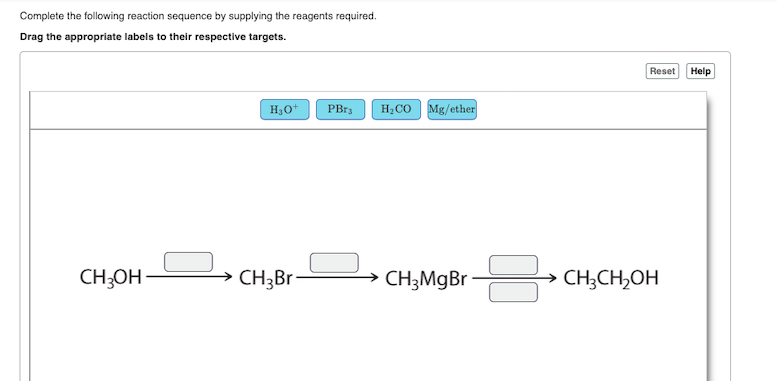10. Complete the following reaction sequence by supplying the reagents required. Complete the following reaction sequence by supplying the reagents required. Drag the appropriate labels to their respective targets. Reset Help H,0 PBr3 H.CO Mg/ether CH3OH > CH3Br D > CH3MgBr - > ChọCH,OH

• ### Complete the following reaction sequence. Complete the following reaction sequence ' 4 pts stucture iii. i...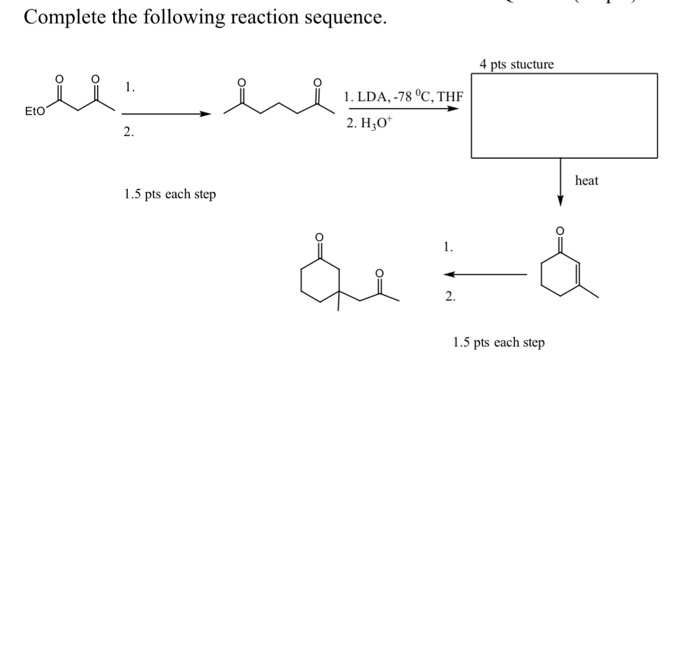Complete the following reaction sequence. Complete the following reaction sequence ' 4 pts stucture iii. i i 1. LDA, -78 °C, THF 2. H30 1. LDA, -78 °C, THE heat 1.5 pts each step de : 1.5 pts each step

• ### may you do this question and show the reaction mechanism 1) Complete the following reaction sequence:...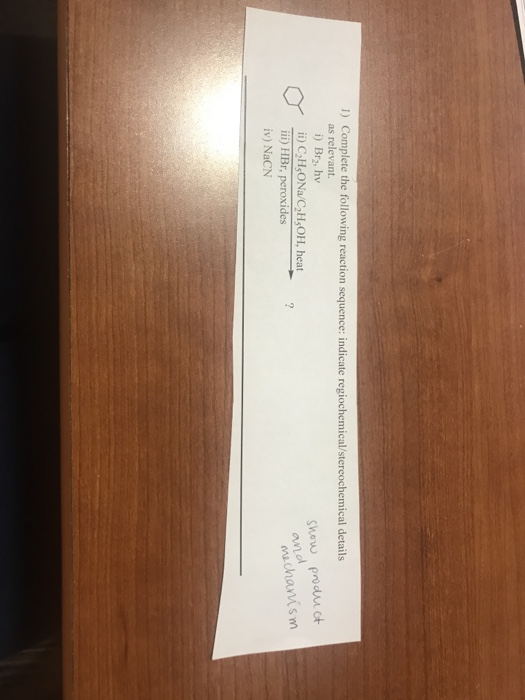may you do this question and show the reaction mechanism 1) Complete the following reaction sequence: indicate regiochemical/stereochemical details as relevant i) Br, hv ii) C,H,ONa/C,H,OH, heat iii) HBr, peroxides iv) NaCN show product and mechanism

• ### EtO, EtOH ai 21. What is the final product of the following reaction sequence? Give structural...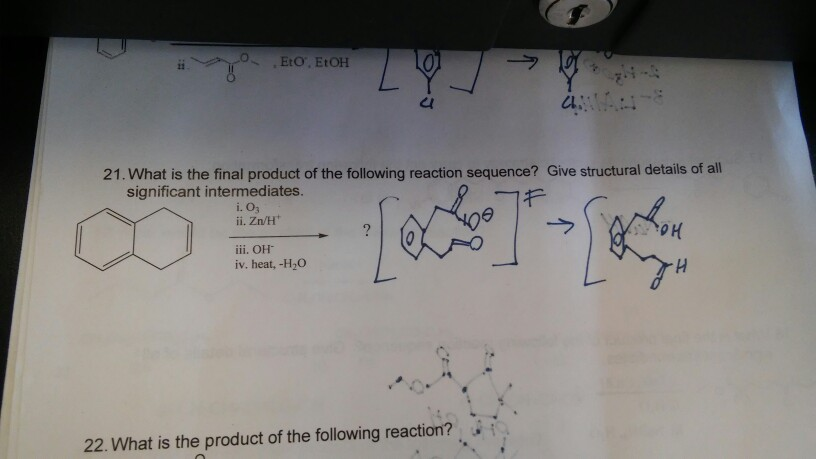EtO, EtOH ai 21. What is the final product of the following reaction sequence? Give structural details of all significant intermediates. ?.03 ii. Zn/H o H 22. What is the product of the following reaction?